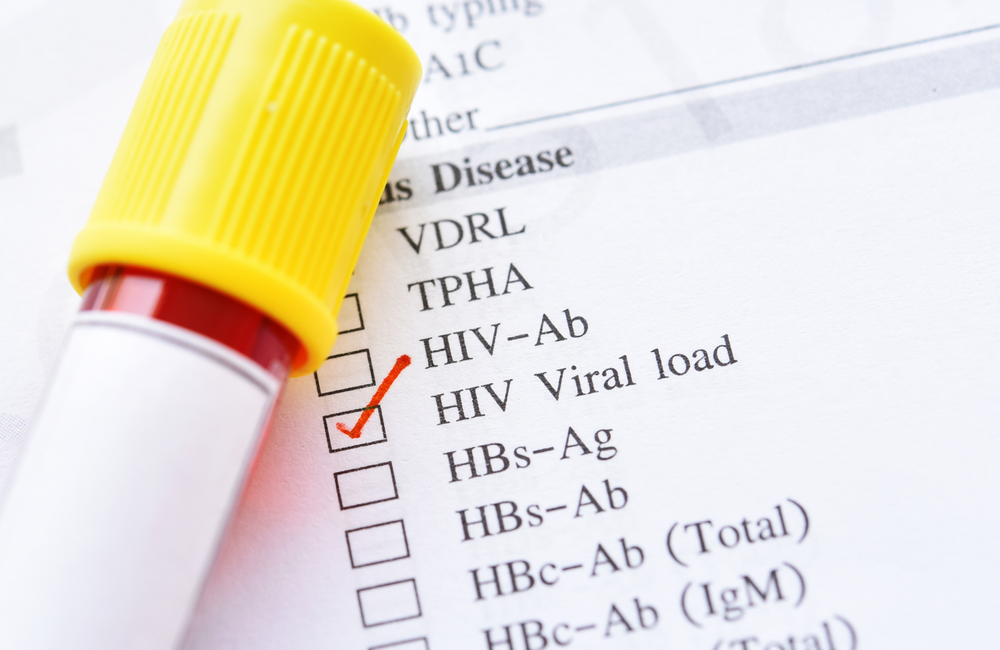# What are logs in viral load results?Image: Jarun Ontakrai/Shutterstock.com

A viral load result is usually described in terms of the number of HIV RNA copies per millilitre of blood (copies/ml). These numbers are often written in a form known as the logarithmic scale, such as 105. This means that the actual number is 10 x 10 x 10 x 10 x 10. Another way of thinking of 105 is as 1 with the decimal point moved five places to the right, the same as 1 plus 5 zeros – 100,000. Viral load variations can also be described using log changes. For example:

• A decrease in viral load from 40,000 to 4000 copies could be described as a one-log decrease, a tenfold decrease, or a decrease of 90%.
• A decrease in viral load from 40,000 to 400 copies could be described as a two-log drop (102), equivalent to a hundredfold fall.
• A decrease in viral load from 40,000 to 40 copies would be a three-log drop (103), equivalent to a thousandfold fall.

Fractions of logs are hard to remember because they do not correspond to round number percentages. For example, a 0.5 log fall in viral load is a 66.6% decrease or a two-thirds fall in viral load. A 1.5 log fall is approximately a 96% reduction.

Scientific notation is a useful way for researchers to present data that include a wide range of values.

Next review date